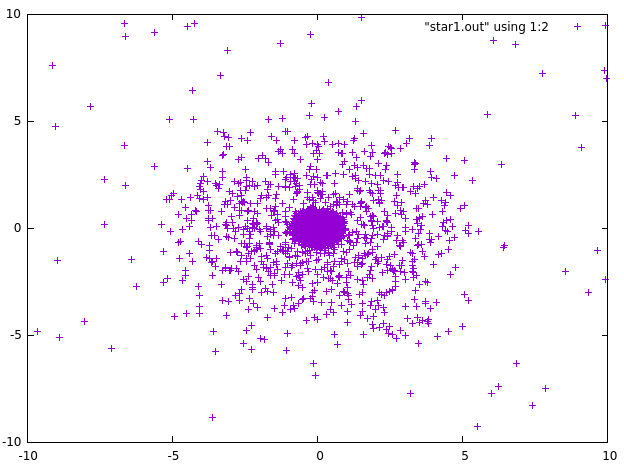too little, too late

home   tsm2

quantum field theory

There are often complaints that quantum field theory is impossible to understand, e.g. CIP repeatedly wrote about buying more and more books about it, but still having trouble comprehending it.

If people have a problem in or with physics, it often is about math and mostly for two reasons:
i) The math used in physics often seems more complicated than what one learns in high school.
ii) The "physicist math" is often ill defined - the physicists often argue, with some justification, that they are way ahead of mathematicians.
Both problems are prominent in QFT: spinors, gauge theory and all that require a lot of advanced math, but some basic concepts, e.g. path integrals, are not even well defined.
My only recommendation is to keep in mind that math is in the end only about re-arranging empty sets and can always be learned given enough time and the proper text books.
Btw I initially learned QFT from Ryder, but there are gentler introductions, e.g. QFT Demystified.

But I suspect that this is not really the problem of CIP et al.
I believe QFT is so hard to understand, because the (classical) models our brain constructs when we learn something are either unavailable or fail completely. I suspect that most people understand even relativity and quantum mechanics by using such models and learning when to switch between them. There is Schroedinger's wave, there are atoms jumping from one state to another, particles tunneling through barriers etc.
I suspect that the need for such classical models is what drives the interpretation debate from Bohm's guiding wave to the splitting of many worlds.
Unfortunately, with QFT the math is pretty much all there is.

But there is some hope for CIP et al. which requires trying something different: lattice field theory (pdf).
A Wick rotation transforms the path integral(s) of QFT into an exercise in statistical mechanics (this trick is not always available, but it works e.g. for QCD). All of a sudden the math is well defined and simple enough that one can simulate QFT on a computer, even at home on a laptop. Btw I would recommend to begin with simulations of the Ising model and the Potts model before moving on to full QCD 8-)
Of course, one needs to keep in mind that "computer time" is very different from real time and the translation from the lattice back to reality can be tricky. But it helps a lot understanding Wilson renormalization, triviality of the Higgs, QCD confinement and other stuff.
And via Boltzmann machines there is even a bridge to neural networks and AI (pdf).

escape velocity

CIP asked a question about the entropy during star formation and I think we got the answer, at least qualitatively; but I would like to understand this better.
So let us begin with this calculation of John Baez, which gets the entropy wrong - it would decrease during star formation, i.e. the gravitational collapse of the matter which makes up the star. What the formula leaves out is the entropy of the outgoing radiation, but I would like to stay in a simple Newtonian model with classical point particles only.
In this case the "missing entropy" must come from the particles with velocities above the escape velocity of the star, which leave the collapsing cluster of particles. (The positions and velocities of the particles are actually not bounded, violating an assumption of this calculation, as he noted at the end of his page.) In other words, the formula John uses can only be an approximation, there is actually no decreasing volume V which encloses all particles and if one defines V considering a sphere which encloses all particles which cannot escape, the number N he uses would not be constant. So how does one really calculate the entropy?
A simpler question would be: If the initial number of particles was N, contained in a volume V, what fraction will escape within a small time interval dt? The Maxwell distribution would tell us the number of particles with velocities above the escape velocity and approximately 1/2 of them would escape, if they are within a distance dt*v from the surface ...
But all this seems a bit unsatisfactory; does anybody have the reference to a full calculation of this problem or do I have to run a computer simulation?

added later: A simple simulation of N=1000 particles, initially contained within a sphere of radius 1 and with zero initial velocity, suggests that after long enough time almost all particles escape to a location outside the initial sphere, due to the simulated gravitational interaction. Of course, my program (quickly cobbled together) could be wrong or inaccurate. The chart below shows the fraction of escaped particles on the y axis after so many time steps on the x axis (I have no explanation for the kink after 500 time steps).The distribution of particles (projected onto a 2d plane) after hundred time steps ...... one can see a "halo" of escaping particles surrounding the majority of particles in the collapsing star.